﻿ Incidence Angle Effect on the Turbulent Flow around a Savonius Wind Rotor

### Incidence Angle Effect on the Turbulent Flow around a Savonius Wind Rotor

Sobhi Frikha, Zied Driss, Hedi Kchaou, Mohamed Salah Abid

American Journal of Energy Research

## Incidence Angle Effect on the Turbulent Flow around a Savonius Wind Rotor

Sobhi Frikha1, Zied Driss1,, Hedi Kchaou1, Mohamed Salah Abid1

1Laboratory of Electro-Mechanic Systems (LASEM), National School of Engineers of Sfax (ENIS), University of Sfax, B.P. 1173, km 3.5 Soukra, 3038 Sfax, Tunisia

### Abstract

This study aims to investigate the effect of the incidence angle on the aerodynamic characteristics of the flow around a Savonius wind rotor. Six configurations with different incidence angles equal to θ=0°, θ=30°, θ=60°, θ=90°, θ=120° and θ=150° were studied. For this, we have developed a numerical simulation using the Computational Fluid Dynamic (CFD) code Fluent. The considered numerical model is based on the resolution of the Navier-Stokes equations in conjunction with the k-ε turbulence model. These equations are solved by a finite volume discretization method. Particularly, we are interested in visualizing the velocity field, the mean velocity, the static pressure, the dynamic pressure, the turbulent kinetic energy, the dissipation rate of the turbulent kinetic energy and the turbulent viscosity. Results confirm that the variation of the incidence angle has an effect on the local characteristics. Our numerical results were compared with those obtained by anterior results. The comparison shows a good agreement and confirms the numerical method.

• Sobhi Frikha, Zied Driss, Hedi Kchaou, Mohamed Salah Abid. Incidence Angle Effect on the Turbulent Flow around a Savonius Wind Rotor. American Journal of Energy Research. Vol. 4, No. 2, 2016, pp 42-53. http://pubs.sciepub.com/ajer/4/2/3
• Frikha, Sobhi, et al. "Incidence Angle Effect on the Turbulent Flow around a Savonius Wind Rotor." American Journal of Energy Research 4.2 (2016): 42-53.
• Frikha, S. , Driss, Z. , Kchaou, H. , & Abid, M. S. (2016). Incidence Angle Effect on the Turbulent Flow around a Savonius Wind Rotor. American Journal of Energy Research, 4(2), 42-53.
• Frikha, Sobhi, Zied Driss, Hedi Kchaou, and Mohamed Salah Abid. "Incidence Angle Effect on the Turbulent Flow around a Savonius Wind Rotor." American Journal of Energy Research 4, no. 2 (2016): 42-53.

 Import into BibTeX Import into EndNote Import into RefMan Import into RefWorks

1234
Prev Next

### 1. Introduction

In this context, we are interested in studying the effect of the incidence angle on the aerodynamic characteristics of the flow around a Savonius wind rotor. For thus, we develop numerical simulations of the turbulent flow using a CFD code.

### 2. Geometric Parameters and Boundary Conditions

The examined Savonius rotor consists of two half-cylinder buckets of diameter d=0.3 m. The overlap is equal to e=72 mm (Figure 1). For the inlet velocity, we take a value of V=9.95 m.s-1, and for the outlet pressure, a value of p=101325 Pa is considered (Figure 2). In this study, we have studied different incidence angles equal to θ=0°, θ=30°, θ=60°, θ=90°, θ=120° and θ=150°. The computational domain effect on the aerodynamic structure around the Savonius wind rotor was previously studied and 40000 cells have been used for the calculations .Download asVeiw figureFigures index
Figure 1. Savonius rotorDownload asVeiw figureFigures index
Figure 2. Boundary conditions

### 3. Numerical Model

Computational fluid dynamic (CFD) simulations are conducted using the commercial CFD code Fluent to study the turbulent flow around a Savonius wind rotor. This code is based on solving Navier-Stokes equations with a finite volume discretization method. Standard k-ε turbulence model has been used for the analysis of the turbulent flow. In fact, this model has been used in different anterior works and satisfactory results were obtained [23, 24].

The mathematical formulation is based on the Navier-Stokes equations. The equations for the conservation of the mass and momentum in the numerical analysis can be written as follows in the Cartesian system.

The continuity equation is:(1)

The momentum equation is: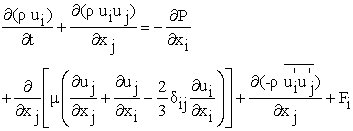(2)

Where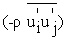is defined by:(3)

In the present work, we have used the k-ε turbulence model. The transport equations for the turbulent kinetic energy k and the dissipation rate of the turbulent kinetic energy ε are written as follows: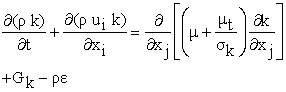(4)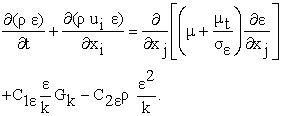(5)

The turbulent viscosity is defined by: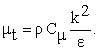(6)

### 4. Numerical Results

4.1. Velocity Field

Figure 3 presents the distribution of the velocity field in the considered computational domain with a zoom around the Savonius rotor for different incidence angles equal to θ=0°, θ=30°, θ=60°, θ=90°, θ=120° and θ=150°. According to these results, the flow appears uniform in the entry of the computational domain. After then, a slowdown of the flow has been noted in the two buckets. Two wakes characteristics of the maximum values of the velocity appear on the two sides of the rotor. A wake characteristic of the minimum value of the velocity occurs downstream of the rotor. A clear asymmetry of the two wakes characteristics of the maximum values of the velocity is shown for an incidence angle equal to θ=0° and θ=30° (Figure 3.a and Figure 3.b). By increasing the incidence angle, the two wake zones become clearly symmetrical for θ=60° and θ=90° (Figure 3.c and Figure 3.d). The dissymmetry resumed again, but in the other direction for θ=120° and θ=150° (Figure 3.e and Figure 3.f). For θ=0°, it has been observed the formation of a recirculation zone in the upper and the lower concave surface of the two buckets. These two zones of recirculation are located downstream of the rotor for θ=0°. However, these two areas are larger for θ=60° and θ=90°. For θ=120°, it has been observed an asymmetry between the two recirculation areas. These two areas become more reduced and disappear for θ=150°. In this case, it has been observed a small area of recirculation on the two surfaces of the two buckets. In addition, it has been noted a formation of a wake characteristic of the minimum values which appear in the form of a band. The width of this band directly depends on the incidence angle. In fact, the maximal width of the band is obtained for θ=60° and θ=120°. The width of this band is reduced for an angle of incidence equal to θ=0° and θ=150°. For the different values of the incidence angles equal to θ=0°, θ=30°, θ=60°, θ=90°, θ=120° and θ=150°, the maximum values of the velocity are equal respectively to V=15.4 m.s-1 (Figure 3.a), V=15.9 m.s-1 (Figure 3.b), V=15.3 m.s-1 (Figure 3.c), V=16.1 m.s-1 (Figure 3.d), V=14.7 m.s-1 (Figure 3.e) and V=14.2 m.s-1 (Figure 3.f). The maximum value is obtained for an angle of incidence equal to θ=90°.

4.2. Mean Velocities

Figure 4 presents the distribution of the mean velocity in the computational domain with a zoom around the Savonius rotor for different incidence angles. According to these results, it has been noted that in the entry of the computational domain, the mean velocity is equal to V=9.95 m.s-1 which is the value already imposed by the boundary conditions. At the Savonius rotor, this value decreases greatly and reaches low values. Two wakes characteristics of the maximum values appear on the two sides of the rotor. Downstream of the rotor, it has been observed a wake characteristic of the minimum values. A dissymmetry of the two wakes characteristics of the maximum values is shown for the incidence angles equal to θ=0°, θ=30° and θ=150° (Figure 4a, Figure 4.b and Figure 4.f). With the increase of the incidence angle, the two wakes become clearly symmetrical for θ=60°, θ=90° and θ=120° (Figure 4.c, Figure 4.d and Figure 4.e). However, it has been noted a formation of a wake characteristic of the minimum values behind the rotor. This wake appears in a form of a band form which depends on the incidence angle. In fact, the maximum width of the band is obtained for θ=30° and θ=90°. For the different values of the incidence angle equal to θ=0°, θ=30°, θ=60°, θ=90°, θ=120° and θ=150°, the maximum values are equal respectively to V=14.5 m.s-1 (Figure 4.a), V=15.2 m.s-1 (Figure 4.b), V=14.8 m.s-1 (Figure 4.c), V=14.9 m.s-1 (Figure 4.d), V=14.7 m.s-1 (Figure 4.e) and V=14.1 m.s-1 (Figure 4.f). Indeed, it has been noted that the maximum value is obtained for an incidence angle equal to θ=30°.

4.3. Static Pressure

Figure 5 presents the distribution of the static pressure in the computational domain with a zoom around the Savonius rotor for different incidence angles. While examining these results, it has been noted that the static pressure is maximum at the rotor. In fact, a compressure zone appears upstream of the rotor and grows on the concave surface of the upper bucket. Behind the rotor, a depression zone has been observed. This zone extends up to the output of the computational domain. By comparing these results between them, it has been noted that the variation of the incidence angle affects the distribution of the static pressure. In fact, for θ=0°, a depression zone has been observed near the Savonius rotor (Figure 5.a). Whereas, for θ=30°, θ=60° and θ=90° (Figures 5.b, Figure 5.c and Figure 5.d), it has been observed a compressure zone on the concave surface of the upper bucket of the rotor. This depression appears again for the incidence angles equal to θ=120° and θ=150° (Figures 5.e and 5.f). For the different values of the incidence angle equal to θ=0°, θ=30°, θ=60°, θ=90°, θ=120° and θ=150°, the maximum values of the static pressure are equal respectively to P=73.9 Pa (Figure 5.a), P=144 Pa (Figure 5.b), P=92 Pa (Figure 5.c), P=20.5 Pa (Figure 5.d), P=39.9 Pa (Figure 5.e) and P=77.2 Pa (Figure 5.f). Indeed, it has been noted that the maximum value of the static pressure is obtained for an incidence angle equal to θ=30°.Download asVeiw figureFigures index
Figure 3. Distribution of the velocity fieldDownload asVeiw figureFigures index
Figure 4. Distribution of the mean velocity
4.4. Dynamic Pressure

Figure 6 presents the distribution of the dynamic pressure in the computational domain with a zoom around the Savonius rotor for different incidence angles. According to these results, it has been noted that in the entry of the computational volume, the dynamic pressure is fairly low. At the Savonius rotor, a decrease in the values of the dynamic pressure has been noted. These values decrease greatly and reach low values. Two compressure zones characteristics of the maximum values appear in the concave and convex surfaces of the upper and the lower buckets. Downstream of the rotor, it has been observed the appearance of a depression zone characteristic of the minimum values. A dissymmetry of the two areas of the wakes characteristics of the maximum values appears for θ=0°, θ=30°, θ=120° and θ=150° (Figure 6.a, Figure 6.b, Figure 6.e and Figure 6.f). With the increase of the incidence angle, the two areas of wake become clearly symmetrical for θ=60° and θ=90° and (Figure 6.c, Figure 6.d and Figure 6.e). However, it has been observed the depression zone behind the rotor. This zone appears in a form of a band which depends directly on the incidence angle. In fact, the maximum width of the band is obtained for θ=120° and θ=150°. For the different values of the incidence angles equal to θ=0°, θ=30°, θ=60°, θ=90°, θ=120° and θ=150°, the maximum values are equal respectively to P=145 Pa (Figure 6.a), P=154 Pa (Figure 6.b), P=144 Pa (Figure 6.c), P=156 Pa (Figure 6.d), P=132 Pa (Figure 6.e) and P=122 Pa (Figure 6.f). The maximum value is obtained for an incidence angle equal to θ=90°.Download asVeiw figureFigures index
Figure 5. Distribution of the static pressureDownload asVeiw figureFigures index
Figure 6. Distribution of the dynamic pressure
4.5. Turbulent kinetic energy

Figure 7 presents the distribution of the turbulent kinetic energy in the computational domain with a zoom around the Savonius rotor for different incidence angles. According to these results, it has been noted that the turbulent kinetic energy is very low in the entry of the computational domain. On the rotor, the turbulent kinetic energy increases, especially on the edges of the two buckets. In fact, it has been observed an occurrence of a wake zone characteristic of the maximum values of the turbulent kinetic energy. For θ=0° (Figure 7.a), this region is located on the outer surface of the upper bucket of the rotor. Whereas, for θ=30° (Figure 7.b), this region appears on the outer surface of the lower bucket. By increasing the incidence angle θ=60° and θ=90° (Figure 7.c and Figure 7.d), it has been noted two wake characteristics of the maximum values. These wakes are located in the external attack side of the upper bucket and on the convex surface of the lower bucket of the Savonius rotor. These two wakes become more extended for an incidence angle equal to θ=120° (Figure 7.e). Away from the rotor, the turbulent kinetic energy becomes very low. For the different values of the incidence angle equal to θ=0°, θ=30°, θ=60°, θ=90°, θ=120° and θ=150°, the maximum values of the turbulent kinetic energy are equal respectively to k=15.9 m2.s-2 (Figure 7.a), k=30 m2.s-2 (Figure 7.b), k=45.1 m2.s-2 (Figure 7.c), k=41.9 m2.s-2 (Figure 7.d), k=22.2 m2.s-2 (Figure 7.e) and k=18.2 m2.s-2 (Figure 7.f). The maximum value of the turbulent kinetic energy is obtained for the incidence angle equal to θ=60°.Download asVeiw figureFigures index
Figure 7. Distribution of the turbulent kinetic energyDownload asVeiw figureFigures index
Figure 8. Distribution of the of the dissipation rate of the turbulent kinetic energy
4.6. Dissipation Rate of the Turbulent Kinetic Energy

Figure 8 presents the distribution of the dissipation rate of the turbulent kinetic energy in the computational domain with a zoom around the Savonius rotor for different incidence angles. According to these results, it has been noted that the wake characteristic of the maximum values is located on the convex surface of the lower bucket of the Savonius rotor as well as in the external attack zone of the upper bucket. Outside this area, a very fast decrease of the dissipation rate has been observed. In addition, these results show that the variation of the incidence angle has a direct effect on the location of the wake zone characteristic of the maximum values. For example, for the incidence angle θ=0°, the wake is located on the convex surface of the upper bucket of the Savonius rotor (Figure 8.a). However, for θ =30°, it has been noted an extension of the wake zone in the external attack zone of the upper bucket (Figure 8.b). also, it has been noted an occurrence of an extended wake on the convex surface of the lower bucket. This wake disappears for θ=60° and θ=90° and it appears on the external attack side of the upper bucket of the rotor. The same fact is presented for the incidence angles θ=120° and θ=150° with the emergence of a second area of wake on the convex surface of the lower bucket. For the different values of the incidence angles equal to θ=0°, θ=30°, θ=60°, θ=90°, θ=120° and θ=150°, the maximum values of the dissipation rate of the turbulent kinetic energy are equal respectively to ε =7330 m2.s-3 (Figure 8.a), ε=14900 m2.s-3 (Figure 8.b), ε=62000 m2.s-3 (Figure 8.c), ε=43500 m2.s-3 (Figure 8.d), ε=19000 m2.s-3 (Figure 8.e) and ε=87200 m2.s-3 (Figure 8.f). The maximum value of the dissipation rate of the turbulent kinetic energy is obtained for the incidence angle equal to θ=150°.Download asVeiw figureFigures index
Figure 9. Distribution of the turbulent viscosity
4.7. Turbulent Viscosity

Figure 9 presents the distribution of the turbulent viscosity in the computational domain with a zoom around the Savonius rotor for different incidence angles. According to these results, it has been noted that the turbulent viscosity is low upstream of the rotor. The values of the turbulent viscosity increase and reach very important values in the two walls above and below the considered computational domain. Also, it has been noted the formation of two wakes characteristics of the maximum values. However, in the Savonius rotor, it has been observed a rapid decrease in the values of the turbulent viscosity. Furthermore, it has been noted that the incidence angle has a direct effect on the distribution of the turbulent viscosity. In fact, for θ=60°, θ=90° and θ=120°, the wakes are located in the two upper and lower wall of the computational domain. The area of the wake extends even more for θ=150°, θ=30° and θ=0°. In these cases, a wake zone characteristic of the minimum values is developed in the rotor and extends downstream of the rotor. When the incidence angle increases, this wake is divided into two asymmetric parts. Indeed, it has been noted that the maximum value of the turbulent viscosity is obtained for θ=90° (Figure 9.d) while the minimum value is obtained for θ=0° (Figure 9.a).Download asVeiw figureFigures index
Figure 10. Evolution of the static torque coefficient CMs

### 5. Comparison with Anterior Results

In this section, we are interested in the study of the influence of the incidence angle θ on the variation of the static torque coefficient CMs of the Savonius rotor. Several incidence angles equal to θ=0°, θ=30°, θ=60°, θ=90°, θ=120° and θ=150° have been examined. Table 1 summarizes the different values of the static torque Ms as well as the static torque coefficient CMs. The variation of the CMs as a function of the incidence angle is presented in figure 10 using the Cartesian and the polar schematization. According to these results, it has been noted that the value of the CMs is quite low for an incidence angle θ=0°. By increasing θ, it has been observed an increase of the static torque coefficient CMs. Indeed, the static torque coefficient reaches a maximum value equal to CMs=0.75 for θ=60°. From this angle, it has been observed a decrease of the value of the CMs, which reaches a minimum value equal to CMs=0.12 for an incidence angle θ=120°. After then, it has been noted an increase and then a decrease in the values of CMs. The results are already expected due to the symmetry presented by the Savonius rotor. The values of the static torque coefficients found for different incidence angles are compared with those found by Menet and Cottier . The profiles of the static torques present the same evolution of the curve. The good agreement confirms the validity of the numerical method.

#### Table 1. CMs values for different incidence anglesDownload asPowerPoint Slide

Veiw figure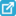View current table in a new window

### 6. Conclusion

Numerical simulation of the turbulent flow around a Savonius wind rotor was investigated for different incidence angles. According to the obtained results, the incidence angle of the Savonius wind rotor has a direct effect on the turbulent flow. Local characteristics such as velocity field, mean velocity, static pressure, dynamic pressure, turbulent kinetic energy, dissipation rate of the turbulent kinetic energy and turbulent viscosity are different from one configuration to another. The variation of the coefficient of the static torque CMs of the Savonius rotor was also studied and numerical results were compared with those obtained by anterior results. A good agreement was obtained and confirmed the numerical method. In the future, we propose to study the effect of the overlap of the buckets on the turbulent flow around the Savonius wind rotor.

### Nomenclature

Cp: coefficient of the power, dimensionless

C: constant of the k-ε turbulence model, dimensionless

C: constant of the k-ε turbulence model, dimensionless

Cμ: constant of the k-ε turbulence model, dimensionless

CMs: static torque coefficient

d: rotor diameter, m

e: bucket thickness, m

Fi: Force components, N

Gk: production term of turbulence, kg.m-1.s-3

k: turbulent kinetic energy, J.kg-1

Ms: static torque

P: pressure, Pa

ui: velocity components, m.s-1

ui’: fluctuating velocity components, m.s-1

ε: dissipation rate of the turbulent kinetic energy, W.kg-1

μ: dynamic viscosity, Pa.s

μt: turbulent viscosity, Pa.s

ρ: density, kg.m-3

σk: constant of the k-ε turbulence model

σε: constant of the k-ε turbulence model

### References

  Kamoji, M.A., Kedare, S.B., Prabhu, S.V., Experimental investigations on single stage modified Savonius rotor, Applied Energy, 86, 1064-1073, 2009.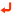In article View Article  Menet, J.L., Bourabaa, N., Increase in the Savonius rotors efficiency via a parametric investigation. European Wind Energy Conference, London, 2004.In article  Blackwell, B.F., Sheldahl, R.E., Feltz, L.V, Wind Tunnel performance data for two and three-bucket Savonius rotor. Journal of Energy, 2-3, 160-164, 1978.In article  Aldos TK, Savonius Rotor Using Swinging Blades as an Augmentation System. Wind Engineer 8 (1984) 214-220.In article  Ushiyama, I., Nagai, H, Optimum design configurations and performances of Savonius rotors. Wind Eng. 12-1, 59-75, 1988.In article  Grinspan, AS, Kumar, PS, Saha, UK, Mahanta, P, Ratnarao, DV, Veda Bhanu, G, Design, development & testing of Savonius wind turbine rotor with twisted blades. Proceedings of international conference on ﬂuid mechanics & ﬂuid power, India, 28, 28-31, 2001.In article  Saha, U.K., Rajkumar, M., On the performance analysis of Savonius rotor with twisted blades, J. Renew. Energy, pp. 960-1481, 2005.In article  Akwa, JV, Júnior, GA, Petry, AP, Discussion on the verification of the overlap ratio influence on performance coefficients of a Savonius wind rotor using computational fluid dynamics. Renewable Energy, 38, 141-149, 2012.In article View Article  Khan, N., Tariq, I. M., Hinchey, M., Masek, V, Performance of Savonius Rotor as Water Current Turbine, Journal of Ocean Technology, 4, N. 2, pp. 27-29, 2009.In article  Rogowski, K., Maronski, R., CFD computation of the Savonius rotor. Journal of Theoretical and Applied Mechanics, 53, no 1, p. 37-45, 2015.In article View Article  Mohamed, M.H., Janiga, G., Thévenin E. Pap, D. Optimization of Savonius turbines using an obstacle shielding the returning blade, Renewable Energy 35, 2618-2626, 2010.In article View Article  Choudhury Bhaskar Jyoti and Saraf Gaurav , Computational Analysis of Flow around a Two-Bladed Savonius Rotor ISESCO Journal of Science and Technology ,Volume 10 - Number 17 - (39-48), 2014.In article  Driss Z., Abid M. S, Numerical Investigation of the Aerodynamic Structure Flow around Savonius Wind Rotor, Science Academy Transactions on Renewable Energy Systems Engineering and Technology, Vol. 2, No. 2, 196-204, 2012.In article  Driss, Z., Mlayeh, O., Driss, D., Maaloul, M., Abid, M. S., Numerical simulation and experimental validation of the turbulent flow around a small incurved Savonius wind rotor. Energy, 2014; 74:506-517.In article View Article  Driss, Z., Mlayeh, O., Driss, S., Driss, D., Maaloul, M., Abid, M. S., Study of the bucket design effect on the turbulent flow around unconventional Savonius wind rotors. Energy, 2015; 89:708-729.In article View Article  Matrawy K.K., Aly Ayman A., Mahrous A. F . Performance Evaluation of Vertical Axis Wind Turbine with a Leading Edge Flap. International Journal of Control, Automation And Systems Vol.3 No.4, 2014.In article  Qasim Ahmed Y., Quadir G.A Hameed., Salih, Obaid Waleed A. and Ziyed Adel Abdalrahman (Performance analysis of a newly designed three frame VAWT having cavity vanes. International Journal Of Engineering And Computer Science ISSN:2319-7242 Volume 3 Issue 10, Page No. 8469-8475, 2014. .In article  D'Alessandro, V., Montelpare, S., Ricci, R., Secchiaroli, A, Unsteady Aerodynamics of a Savonius wind rotor: a new computational approach for the simulation of energy performance, Energy 35, 3349-3363, 2011.In article View Article  Dobreva, I, Massouh F., CFD and PIV investigation of unsteady flow through Savonius wind turbine, Energy Procedia 6, 711-720, 2011.In article View Article  Kacprzak, K., Liskiewicz, G., Sobczak, K , Numerical investigation of conventional and modified Savonius wind turbines, Renewable Energy 60, 578-585, 2013.In article View Article  Zhou, T., Rempfer, D., Numerical study of detailed flow field and performance of Savonius wind turbines, Renewable Energy 51, 373-381, 2013.In article View Article  Roy, S., Saha U.K, Review on the numerical investigations into the design and development of Savonius wind rotors, Renewable and Sustainable Energy Reviews 24, 73-83, 2013.In article View Article  Frikha, S., Driss, Z., Hagui, M. A, Computational study of the diffuser angle effect in the design of a waste heat recovery system for oil field cabins. Energy, 84, 219-238, 2015a.In article View Article  Frikha, S., Driss, Z., Hagui, M. A, Computational Study of the Turbulent Flow inside a Waste Heat Recovery System with a 25° inclined Angle Diffuser. American Journal of Mechanical Engineering, 3(3A), 1-8, 2015b.In article  Menet, J.L., Cottier, F., Étude paramétrique du comportement aérodynamique d'une éolienne lente à axe vertical de type Savonius, 16è Congrès Français de Mécanique, Nice, 2003.In article